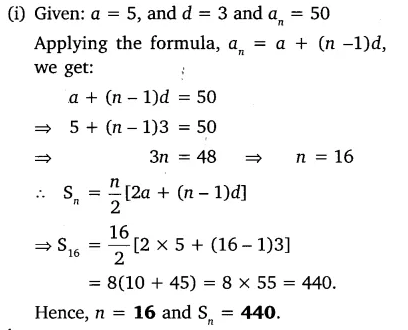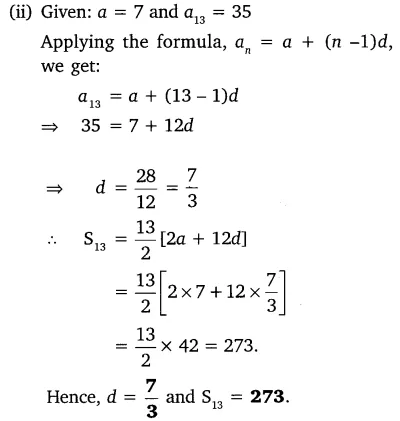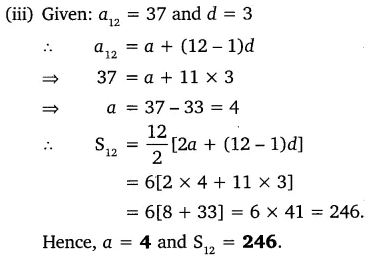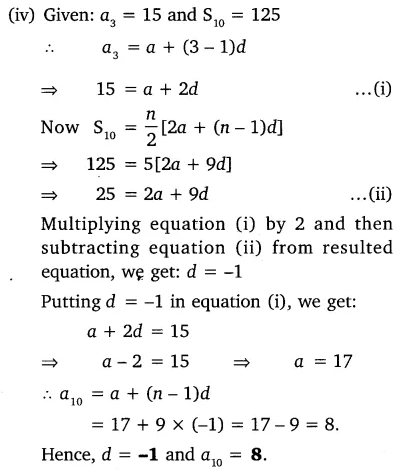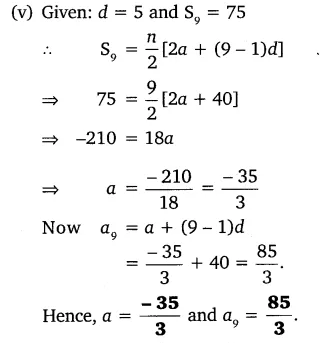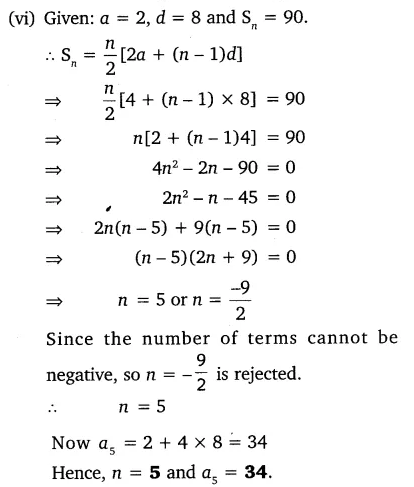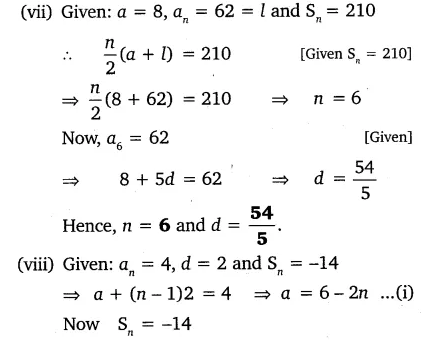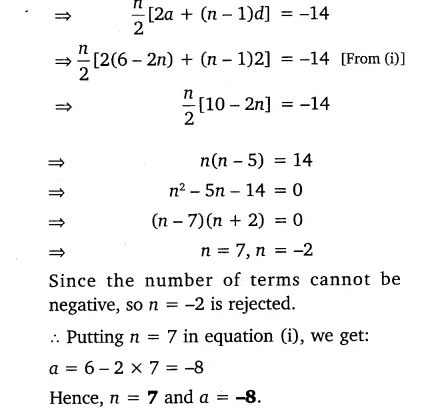# In an AP:(i) given a = 5, d = 3, an = 50, find n and Sn

In an AP:
(i) given a = 5, d = 3, an = 50, find n and Sn.
(ii) given a = 7, a13 = 35, find d and S13.
(iii) given a12 = 37, d = 3, find a and S12.
(iv) given a3 = -15, S10 = 125, find d and a10.
(v) given d = 5, S9 = 75, find a and a9.
(vi) given a = 2, d = 8, Sn = 90, find n and an.
(vii) given a = 8, an = 62, Sn = 210, find n and d.
(viii) given an = 4, d = 2, Sn = -14, find n and a.
(ix) given a = 3, n = 8, S = 192, find d.
(x) given l = 28, S = 144, and there are total 9 terms. Find a.